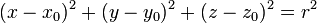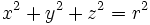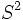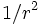# 2-sphere

## Definition

A 2-sphere in$\R^3$ is the set of all points having a fixed distance (termed the radius) from a fixed point (termed the center). The 2-sphere is a particular case of the more general notion of sphere, which makes sense in all dimensions.

The radius is denoted as$r$.

## Equational descriptions

### Cartesian equation

The 2-sphere with center$(x_0, y_0, z_0)$ and radius$r$ has the following Cartesian equation:$(x-x_0)^2 + (y-y_0)^2 + (z - z_0)^2 = r^2$

since the center can be translated to the origin, we can consider the sphere centered at the origin, whose equation is:$x^2 + y^2 + z^2 = r^2$

## Abstract structure

All 2-spheres are the same upto similarity, and the 2-sphere is thus uniquely determined topologically, differentially, and upto isometry (upto scaling factor by a constant).

The abstract 2-sphere is denoted as$S^2$.

## Structure and symmetry

The 2-sphere is a quotient space, viz it occurs as the quotient of a Lie group by a subgroup. Thus, the group of isometries is transitive on all points.

## Curvatures

Every point on the 2-sphere is an umbilic point, viz the curvature in all directions at each point is equal. In fact, the curvature in any direction is$1/r$, hence we have:

• The Gaussian curvature is$1/r^2$
• The mean curvature is$2/r$

## Properties

The metric on the 2-sphere has constant Gaussian curvature (The two-dimensional version of sectional curvature) everywhere.

Given any two points on the 2-sphere, there is an isometry taking one to the other.

## Related theorems

### Liebmann's theorem

This provides an instance of application of the following theorem: Liebmann's theorem

We saw that the mean curvature of the 2-sphere is$2/r$ where$r$ is the radius. Liebmann's theorem establishes a converse of sorts: any differentiable, closed and convex surface whose mean curvature is constant, must be a 2-sphere.